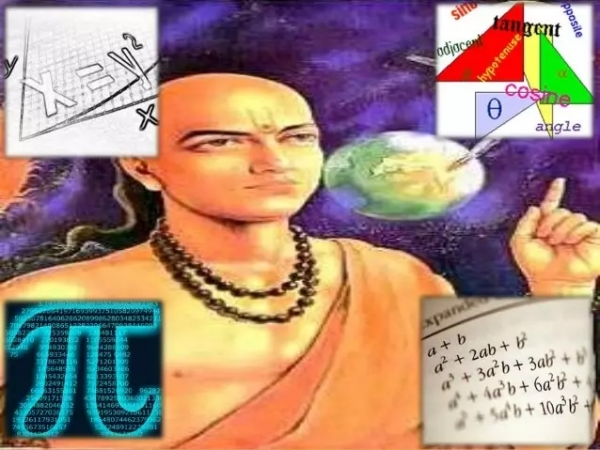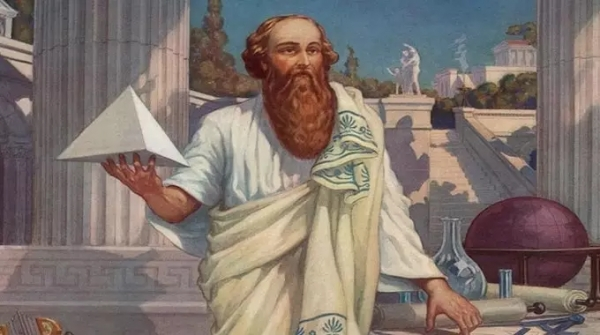# Who were the early Mathematicians?

Mathematics is the most important subject since ages like from the times of Mesopotamians, Egyptians, and Greek people. It has been understood that the initial stages of Mathematics have evolved since 35000 BC where they had first notched the tally bones. Africans, Sumerians, Egyptians, Babylonians, Chinese, Indians and Greeks were all the early Mathematicians.## Indian Scholars

It was understood that during 1200 BC to the 18th century, many scholars such as Aryabhatta, Brahmagupta, Bhaskaracharya, Mahavira, Madhava, Nilakantha Somayaji etc. have contributed crucial theorems of Mathematics starting from the invention of Zero to negative numbers, arithmetic, algebra, trigonometry, geometry, and many astronomical calculations.

Many of those Mathematical principles were made in poetry form in Sanskrit and made easy for the students to memorize in a rhythm. Ancient Gurukulas were the holy schools which used to inherit the students with all sorts of knowledge in all the extensive courses of Education for free.

## Aryabhatta

He was a great renowned Indian Scholar who is a Mathematician cum astronomer, that lived during 476-550 CE. He is famously known as the “inventor of zero” who has done many significant contributions in the field of Mathematics. His works, the Aryabhatiya and the Arya-Siddhanta were the bibles of then mathematicians. Aryabhatta has also worked on the approximation of Pi.

## Pythagoras

Pythagoras who lived during 570-495 BC was the Greek Mathematician and Philosopher. The famous Pythagorean theorem which states that in a right-angled triangle the length of the hypotenuse is equal to the sum of the two sides, which he invented was named after him. He founded the Pythagorean School of Mathematics in Cortona, Italy.## Euler

One of the most profound early Mathematicians was Leonhard Euler who lived in 1707-1783 has published more than 900 books during his time. The famous Euler’s formula and Euler’s number in Mathematics were named after him. The present Sudoku which is widely played by many to improve their skills was formulated by Euler initially.

## Gauss

A Significant Mathematician during 1777 – 1855 was Carl Friedrich Gauss who is famous for his contributions to astronomical mathematics. He discovered that errors in astronomical measurements lead to the bell curve, which was later understood as the Gaussian distribution curve.

There were many renowned scholars of Mathematics of those early times from many different countries, all over the world.